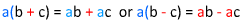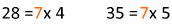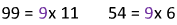# Factors and the Distributive Property

What is the distributive property?Looking at the property with symbols might seem a little confusing at first, so let's look at the distributive property as an area model.Now, let's put this diagram into math terms using the property:The distributive property is a useful way to look at problems in a new way. The distributive property helps us to rewrite expressions and still get the same answer. Check out a couple of examples.

Example 1: Take a look at this expression: 28 + 35.

What do 28 and 35 have in common? Seven is a factor of both of them.So this means that 28 + 35 = (7 x 4) + (7 x 5).

Now, we bring in the distributive property.

We can take the 7 out of both terms and be left with the 4 and 5.The answer to 28 + 35 and the answer to 7(4 + 5) is 63. The answer is the same to both. However, you might find that getting to the answer in the second form is easier. So we can use the distributive property to rewrite expressions with common factors to help simplify the work.

Example 2: Take a look at a new expression: 99 - 54.

What do 99 and 54 have in common? Nine is a factor of both of them.So this means that 99 - 54 = (9 x 11) - (9 x 6).

Now, we will use the distributive property again.

We can take the 9 out of both terms and be left with the 11 and 6.The answer to 99 - 54 is the same as the answer to 9(11-6). Both have an answer of 45. Again, using the distributive, we can rewrite the expression. Rewriting the expression can sometimes help us to solve the question more easily or shed light on different features of the problem.

Let's extend the distributive property to include variables. We will start with an area model again.This shows us that 8(x + 13) = 8x + 8(13) = 8x +104. So 8(x + 13) is the same as 8x + 104.

Here are a few more examples:Let's Review: The distributive property is a great way to rewrite expressions in order to solve them in a different way. We can distribute, or multiply each number in the parentheses by the number outside the parentheses.

5(x + y) = 5(x) + 5(y) = 5x + 5y

We also use the distributive property to solve in the opposite order.

99 + 63 = 9 x 11 + 9 x 7 = 9(11 + 7) = 9(18)

Not matter how you use it, the distributive property helps us the rewrite expressions in a new equivalent form that can be used to help us to solve.